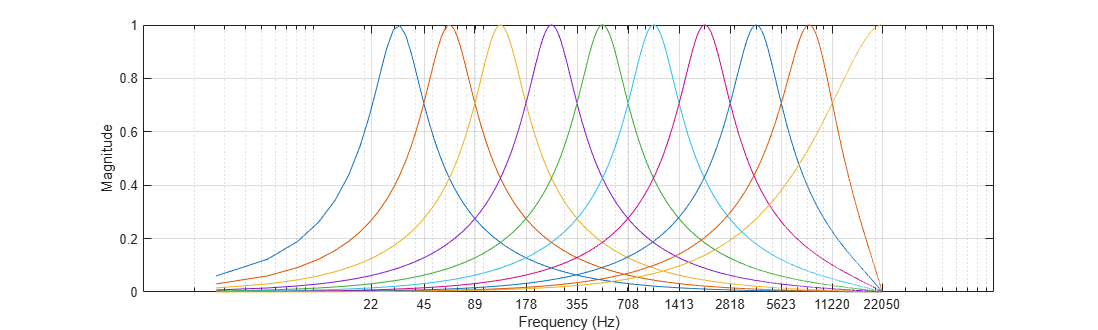# getBandedgeFrequencies

Get filter bandedges

## Syntax

``bandEdges = getBandedgeFrequencies(obj)``
``[bandEdges,centerFrequencies] = getBandedgeFrequencies(obj)``

## Description

example

````bandEdges = getBandedgeFrequencies(obj)` returns the bandedge frequencies of the filters designed by `obj`. If there are M filters, then there are M center frequencies and M+1 band edge frequencies.```
````[bandEdges,centerFrequencies] = getBandedgeFrequencies(obj)` returns the center frequencies of the filters designed by `obj`.```

## Examples

collapse all

Create a default `octaveFilterBank` object.

`octFiltBank = octaveFilterBank;`

Call `getBandedgeFrequencies` to return a vector of bandedge frequencies.

`bE = getBandedgeFrequencies(octFiltBank)`
```bE = 1×11 104 × 0.0022 0.0045 0.0089 0.0178 0.0355 0.0708 0.1413 0.2818 0.5623 1.1220 2.2050 ```

Call `freqz` to get the frequency response of the filter bank. Plot the magnitude frequency response. Use the bandedge frequencies to label the frequency axis.

```[H,f] = freqz(octFiltBank); semilogx(f,abs(H)) xticks(round(bE)) xlabel('Frequency (Hz)') ylabel('Magnitude') grid on h = gcf; set(h,'Position',[h.Position(1) h.Position(2) h.Position(3)*2 h.Position(4)])```## Input Arguments

collapse all

Object to get filter information from, specified as an object of `octaveFilterBank`.

## Output Arguments

collapse all

Bandedges of filters designed by `obj` in Hz, returned as a row vector.

Data Types: `double` | `single`

Center frequencies of filters designed by `obj` in Hz, returned as a row vector.

Data Types: `double` | `single`